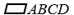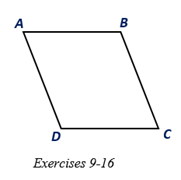Chapter 4.1, Problem 9E### Elementary Geometry for College St...

6th Edition
Daniel C. Alexander + 1 other
ISBN: 9781285195698

#### Solutions

Chapter
Section### Elementary Geometry for College St...

6th Edition
Daniel C. Alexander + 1 other
ISBN: 9781285195698
Textbook Problem
1 views

# Given that A B = 3 x + 2 , B C = 4 x + 1 , and C D = 5 x - 2 , find the length of each side of.To determine

To find:

The length of each side of ABCD.

Explanation

Given:

Given that AB=3x+2, BC=4x+1, and CD=5x-2, and the diagram as shown below.

Corollary:

The opposite sides of a parallelogram are congruent.

Calculation:

In the given parallelogram ABCD, given that AB=3x+2, BC=4x+1, and CD=5x-2.

As per the corollary that the opposite sides of a parallelogram are congruent, the opposite sides B=DC, and AD=BC.

Since AB=DC

3x+2=5x-2

3x-5x=-2-2

-2x=-4

x=-4-2=2

### Still sussing out bartleby?

Check out a sample textbook solution.

See a sample solution

#### The Solution to Your Study Problems

Bartleby provides explanations to thousands of textbook problems written by our experts, many with advanced degrees!

Get Started

#### 2xx+1+4x+1

Applied Calculus for the Managerial, Life, and Social Sciences: A Brief Approach

#### Sketch the graphs of the equations in Exercises 512. xy=4

Finite Mathematics and Applied Calculus (MindTap Course List)

#### If f is continuous on [0, 1], prove that 01f(x)dx=01f(1x)dx

Single Variable Calculus: Early Transcendentals, Volume I

#### True or False: If F is conservative, then curl F = 0.

Study Guide for Stewart's Multivariable Calculus, 8th